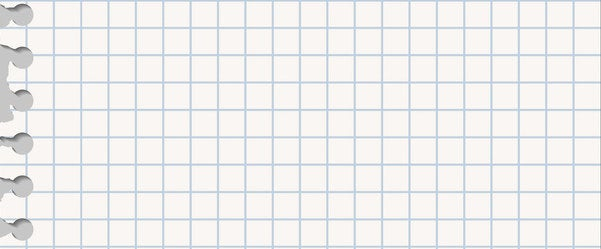1. Which of these is a ray?Getty Images
Correct!
Wrong!

A

A ray starts at one point and continues in one direction infinitely.Via BuzzFeed
2. Can you identify the set of parallel lines?Getty Images
Correct!
Wrong!

C

Parallel lines do not intersect.Via BuzzFeed
3. Which of these is a trapezoid?Getty Images
Correct!
Wrong!

C

Trapezoids have one pair of opposite, parallel sides.Via Getty Images
4. Which formula should you use to find the area of a circle?Getty Images
Correct!
Wrong!

B

The area of a circle can be found by multiplying pi by the square of the radius.

5. Which of these lines depicts the radius of a circle?Getty Images
Correct!
Wrong!

A

The radius starts at the center of a circle and ends at its perimeter.Via BuzzFeed
6.BuzzFeed
Correct!
Wrong!

This shape has acute angles.

The shape has obtuse angles, because they are all greater than 90º.

7. Which of these is an obtuse angle?Getty Images
Correct!
Wrong!

A

An obtuse angle is any angle between 90° and 180º.8. What are supplementary angles?Getty Images
Correct!
Wrong!

Supplementary angles add up to 180º.

9.BuzzFeed
Correct!
Wrong!

70º

These two angles are complementary, meaning their sum equals 90º. 90 minus 20 is 70, so angle X = 70º.

10.BuzzFeed
Correct!
Wrong!

55º

These two angles are supplementary angles, meaning they add up to 180º. 180 minus 125 is 55, so Z = 55º.Via BuzzFeed
11.BuzzFeed
Correct!
Wrong!

C

The hypotenuse is the longest side of a right triangle.Via BuzzFeed
12. And finally, is the following statement true or false?

All squares are rectangles, but not all rectangles are squares.Getty Images
Correct!
Wrong!

True

A rectangle has four right angles and the opposite sides are equal — all of which is true for squares. But every side of a square must be equilateral, which is not true of rectangles.